ISSN: 0970-938X (Print) | 0976-1683 (Electronic)

# Biomedical Research

An International Journal of Medical Sciences

Research Article - Biomedical Research (2017) Volume 28, Issue 16

## A hybrid segmentation approach for detection and classification of skin cancer

Akila Victor* and Muhammad Rukunuddin Ghalib

Vellore Institute of Technology, Vellore, Tamil Nadu, India

*Corresponding Author:
Akila Victor
Vellore Institute of Technology
Tamil Nadu, India

Accepted date: June 2, 2017

Visit for more related articles at Biomedical Research

### Abstract

Advancement in Computer Aided Diagnostic system (CAD) enhances the detection and classification of domain experts and reduces the time rapidly for them. The CAD systems can be used in hospitals as an alternate method. The objective of the paper is to present the effectiveness of the detection and classification of skin cancer. The proposed methodology concentrates on comparing the median filter and Adaptive Median Filter (AMF) and suggesting on one, the segmentation can be done by a hybrid approach where the marker controlled watershed algorithm is fused with the active contour algorithm, the feature extraction is done with the help of basic statistical methods and the Grey Level Co- Occurrence Matrix (GLCM) with the Support Vector Machine (SVM) for classification. SVM is used to classify the input as cancerous or not. The experiment is carried out on 250 images consists of 100 normal images and 150 abnormal images (benign and melanoma images) from a skin dataset. The classification accuracy shows 94% after the classification.

## Keywords

Adaptive median filter, Active contour, Marker control watershed, Grey level co-occurrence matrix (GLCM), Support vector machine (SVM).

## Introduction

The computer aided diagnostic system is something which helps in the experts to process the system pretty quickly. The CAD system helps in assisting the physician to make decision so it helps in decision support system. The analysis can be done in a very short time using CAD systems. CAD systems are built in such a way that they can detect a melanoma image. Cancer is a dreadful disease that occurs most commonly nowadays. It can be treated but still the treatment is a very challenging one. The dreadful disease can occur any time right from kids to grown up. We are discussing on the various possibility to detect the tumour earlier. Skin cancer can be of various types where melanoma is a life threatening disease. The melanoma and benign are two classes where melanoma meaning the cancerous one and the other one is not. Skin cancer can have various stages. It can occur from a mole, exposure to sunlight and hereditary as well. This proposed method can be using the adaptive median filter and the comparison on the adaptive and median filter are also expressed. The segmentation can be a hybrid method followed by a feature extraction and then finally the classification procedure to determine the accuracy.

## Related Work

There are various filtering techniques discussed on skin cancer detection like image scaling, color space transformation  and the hair removal algorithm of dull razor software can also be included  that removes the hair in an image. The contrast enhancement can also apply as a filter  which helps in improving the contrast. Finding the region of interest is a very important role to play with. And it can be using Gradient Vector Flow (GVF), level set methods, adaptive thresholding, adaptive snake, EM level set,  Fuzzy based split and merge. One another segmentation which helps in maximum entropy threshold. The watershed algorithm which is used mostly for segmentation is explained in [5,6]. The feature extraction can explain on GLCM,  ABCD features [8-11]. Gabor filters and the other methods to extract the features explained [12-16]. The extracted features are subjected to classification. The accuracy plays a vital role in medical image processing. The accuracy obtained are calculated based on the various metrics of classification from algorithms like artificial neural networks [17,18] support vector machine, K-nearest neighbour [19,20] and the back propagation algorithm.

This paper is organised as follows: section 2 presents the related works and section 3 explains the proposed methodology describing the pre-processing of skin cancer images, the hybrid segmentation methodology, the feature extraction on the segmented image and finally the classification done by four different classifiers. Section 4 describes the performance evaluation and section 5 explains the obtained results that are found by the above steps. And finally section 6 draws a conclusion and gives a hint for the future work to be done.

## Materials and Methods

The proposed methodology in Figure 1 explains the overview of the skin cancer detection and classification. The input image is taken and that is subjected to the pre-processing phase. Preprocessing is removing the noise. The medical imaging works well for the noise free images. The pre-processing uses the adaptive median filter to remove the noise. Then the median filter and the adaptive median filter are compared and the values based on the metrics are calculated. The various metrics are compared for both the median filter and the adaptive median filter. The adaptive median filtered image is fed as an input to the segmentation phase. The segmentation uses the hybrid method of both the active contour and the marker control watershed algorithm. The segmentation using active contour is extracted and the output of that is fed as an input to marker control watershed algorithm. Now the segmented image is extracted for GLCM features and the basic statistical method. Then the features are fed as an input to the support vector machine to examine the accuracy.

Figure 1: The proposed methodology for detecting and classification of skin cancer.

Image enhancement

The first step is to acquire an image. The acquired image is then subjected to pre-processing. Pre-processing is the step which is used to enhance an image. The enhancement stage is used to filter the noise and brighten the image. The error rate gets reduced and the pixel intensities are improved by using the noise filters. There are various noise filters like box filter, weighted average filter, median filter, mean filter, mode filter and various other filters. Our proposed method uses the median filter and the adaptive median filter for pre-processing and the comparative results are discussed here.

Median filter

Median filter is one of the best used filters for pre-processing medical images. The median filter is a filter which helps to remove noise and brighten the image without noise factor. The median filter operations can be by using masks. Firstly the image is arranged in ascending order and then the median of the image is found and replaced by the original pixel intensity values.

Adaptive median filter

The adaptive median filter is an advanced version of median filter. The adaptive median filter as the name suggests uses the adaptive mask which differs from application to application. The adaptive median filter uses the following algorithm.

Stage 1:

H1=Fmedian-Fmin

H2=Fmedian-Fmax

If F1>0 and F2<0 go to stage 2

Else increase the filter size

If filter size = Smax repeat stage 1

Else output Fmedian

Stage 2:

G1=Fxy-Fmin

G2=Fxy-Fmax

If G1>0 and G2<0, display Fxy

Else display Fmedian

Sxy is the support of the filter centered at x, y.

Fmedian is nothing but the median of gray levels at Sxy. Fmin is the minimum gray level at Sxy

Fmax, Fmin are the minimum and the maximum gray levels at Sxy.

Fxy is simply the gray level at coordinates x, y.

Smax is the maximum size allowed size of Sxy.

The adaptive median filter algorithm discusses about 2 stages in stage 1 the mask is first determined adaptively. Then the difference of median and the minimum gray level value is found. Then the difference of median and the maximum gray level value is found and they are compared, if the former is greater than zero and the latter is lesser than zero go to stage 2. There the difference between the centre pixel and the minimum intensity level pixel is found and then the difference between the centre pixel and the maximum intensity pixel is found and then if the former is greater than zero and the latter is less than zero the value at Fxy is assumed as the final value. Else the size of the mask is increased if the mask is less then repeats the stage 1 else replace the value with the median of the mask.

Comparison of median filter and the adaptive median filter

The median filter and the adaptive median filter are good at their own performance. We have tested with the dataset that we have on both the median filter and the adaptive median filter. The adaptive median filter has given a better result when compared to that of a median filter as far as our dataset is concerned. The adaptive median filter and the median filter are tested with 75 images. The samples of the tested images are displayed in Table 1. The comparative study of the filters can be represented by means of a graph as well. The adaptive median filter therefore is used to remove the noise and enhance the image.

Adaptive median filter Median filter
Input Image PSNR SNR MSE PSNR SNR MSE
1 32.8177 28.2152 52.1294 28.411 23.8085 52.54
2 34.169 29.8256 24.2677 27.4568 23.1133 26.5067
3 31.9478 27.7946 26.7344 26.5975 22.4442 28.8753
4 30.2266 26.05 30.4705 24.5032 20.3266 32.3335
5 32.6065 25.9556 55.2646 29.5707 22.9198 55.4294
6 29.6225 25.9313 41.8588 23.4473 19.7562 43.4436
7 30.0273 26.6006 34.3551 23.6879 20.2613 36.1168
8 29.9059 25.4595 38.0873 23.814 19.3676 39.2061
9 30.329 26.522 38.6791 23.9909 20.1839 40.121
10 31.44659 25.8673 24.4702 25.3576 19.7589 26.2431
11 29.1108 25.1612 30.7278 23.658 19.7083 32.4846
12 28.075 25.9492 28.0483 21.8482 19.7224 31.7959
13 29.085 25.049 53.3695 23.2945 19.2585 53.2685
14 33.8174 28.9424 23.4498 27.4732 22.5982 25.6438
15 34.2554 28.9412 22.7558 27.3259 22.0117 24.3775
16 32.1612 27.9163 31.2433 25.8704 21.6254 32.4126

Table 1: Comparison of the metrics on pre-processing such as PSNR, SNR and MSE between median filter and adaptive median filter.

Segmentation

The segmentation is the process by which the entire image is divided into multiple parts. The segmentation helps in identifying the region of interest. Here we use the active contour segmentation algorithm and marker control watershed algorithm for the process of segmentation. The pre-processed image is fed into the active contour algorithm as this algorithm supports noise free images, and the output of the active contour is fed as an input to the marker control watershed algorithm. And the features are then extracted from the segmented image.

Active contour

The enhanced image is fed as input to the segmentation phase. There are three important steps in this phase. The first step is to represent object boundary or any parametric curve. The second step focuses on energy calculation and that energy should be minimised. Step three starts at a point and it moves towards a certain boundary and then it shrinks.

The contour point is defined as

C(s)=(A (s), B (s)) → (4)

The energy can be calculated as

Energy=Internal energy+External energy+Constraint energy

The energy is calculated as the sum of internal energy, external energy and constraint energy. Internal energy is of elastic energy and blending energy.

Internal energy=Elastic energy+Blending energy

Elastic energy=1/2 ∫ α (s) mod C(s)2 ds → (5)

Blending energy=1/2 ∫ β (s) mod css2 ds → (6)

By using Equations 4-6 we compute the energy by using the elastic and bending property the elastic property gives a stiff image whereas the blending energy gives a smooth region. Internal energy is obtained by adding elastic energy and blending energy. The next calculation is the external energy or the boundary calculations. They are represented as follows

External energy=∫0 1Image C (s) ds → (7)

The final active contour can be represented as the sum of the internal energy, external energy and the constraint energy as discussed in the above equations.

Marker controlled watershed

The obtained output is fed into the next segmentation phase. The gradient magnitude is computed. The background markers and the foreground objects are detected using the opening followed by the reconstruction phase and then the closing is performed. The watershed ridges are identified. Colored watershed labels are marked and the region of interest is extracted by means of marked watersheds.

Feature extraction

The feature extraction is the next phase of the detection and classification methodology. The features are extracted by the segmented image and then the feature extraction is done by combining the GLCM and normal statistical method.

Normal statistical method

The basic statistical methods are used to calculate the area, mean, variance and standard deviation in the features. The features calculation is tabulated according to the below Equations 1-4. Area is calculated as the whole segmented image. Mean is the whole summed up divided by total and variance and standard deviation is calculated along with the GLCM features extraction.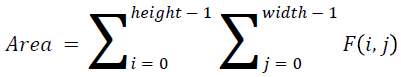→ (8)→ (9)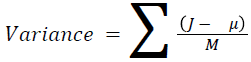→ (10)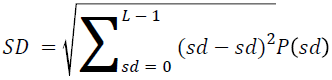→ (11)

GLCM

GLCM is a second order feature, it is calculated at a distance d with an angle θ. The input is arranged as its corresponding cooccurrence matrix which has nearly 20 features to be considered the features are entropy, energy, contrast, correlation, homogeneity, cluster prominence, cluster shadowing, dissimilarity, maximum probability, sum of squares, sum average, sum variance, sum entropy, difference variance, difference entropy, etc. The formula that is used to calculate the above features are as listed below.

Homogeneity

They contain the homogenous that is the same gray level values→ (12)

Contrast

The measure of local intensity variation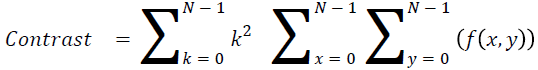→ (13)

Local homogeneity (LH)

Relatively higher value will be obtained out of this feature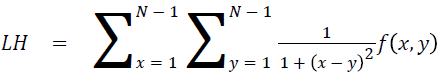→ (14)

Entropy

It is actually representing the disorders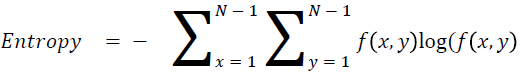→ (15)

Correlation

The measure of intensity dependence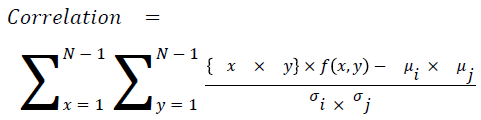→ (16)

Sum of square: Variance

It is the average of the mean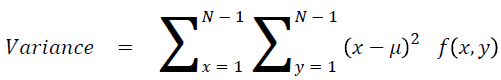→ (17)

Sum average

The sum of average is something where the total value is found.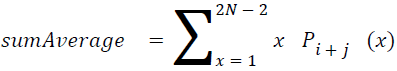→ (18)

Sum entropy

The sum entropy is as follows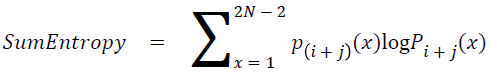→ (19)

Difference entropy

The difference entropy is as follows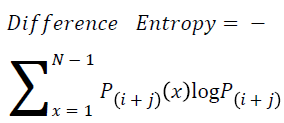→ (20)

Cluster shade

The cluster shade is as follows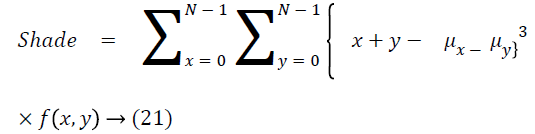Cluster prominence

The cluster prominence is as follows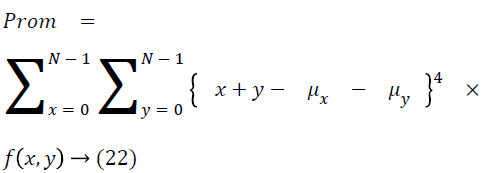Thus the Equations 4-22 explain how the features can be extracted from the segmented image and how the features can be fed into the classifiers.

Classification

The classification is the final stage which classifies into benign or melanoma image. Benign meaning a normal image and melanoma the cancerous image. Support Vector Machine (SVM) is the classifier used here where it uses the hyper plane to classify and misclassify the classes. Two classes defined one is class 1 the normal one, class 2 the cancerous one. The features are fed into the classifier and the classification accuracy obtained is 94%.

## Results and Observations

The results and discussion of the hybrid segmentation algorithm that helps to detect and classify the skin cancer images are discussed in this session. This session explains the result of all the four phases preprocessing, segmentation, feature extraction and classification.

Preprocessing

The noise removal stage or the first phase of any medical image processing is the preprocessing phase. Here the preprocessing phase uses both the median filter and the adaptive median filter and then both are eventually compared. The below Table 1 explains the comparison of both the adaptive median filter and the median filter.

The Table 1 tabulates the value of the metrics of pre-processing such as Peak Signal to Noise Ratio (PSNR), Signal to Noise Ratio (SNR) and Mean Square Error (MSE). The metrics are calculated as follows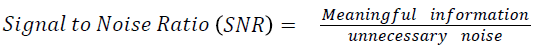→ (1)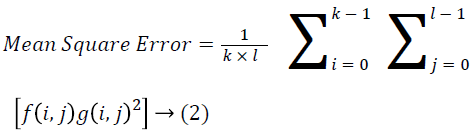→ (2)

k × l-noise free order of a monochrome image I

J-Noisy approximation

Peak signal to noise ratio (PSNR) =20 × log10 (MPV)-10 × log10 (MSE) → (3)

MPV: Maximum Possible Pixel Value

MSE: Mean Square Error

The Equations 1-3 shows how the metrics of pre-processing are calculated based on the equation that is discussed. They can also be expressed in form of a comparison graph.

Thus Figures 2-4 can very well show that the comparisons were made and they evidently show that the adaptive median filter has an upper hand over a median filter. And hence the dataset works well for the adaptive median filter rather than the median filter.

Segmentation

The segmentation can be done for the preprocessed image. The preprocessed image can be fed as an input to the segmentation phase. The segmentation uses the active contour algorithm and the marker control watershed algorithm. The active contour uses three important steps: the first one is to identify the object boundary, the second one is to compute the energy that is the internal energy, external energy and the constraint energy. Then the final object shrinks and the segmented portion is obtained. The output of that is fed as an input to the marker controlled phase. The gradient magnitude is calculated. The foreground image is obtained by opening followed by the reconstruction phase. Then the background values are marked. The watershed transform is used to calculate the ridges and the final result is visualized. The segmentation result is given in Figure 5 after all the segmentation process applied.

Feature extraction

The feature extraction helps to extract the features. There are 27 features extracted from the segmented image. The various features play a very vital role since the features are the essential elements that mostly determine the accuracy in an image. The feature extraction can be called as the heart of the process. The extracted features are then fed to the classification phase so as to classify the class 1 normal and the class 2 melanoma images. The classification and the misclassification are formed by the support vector machine which is described in the below equation and the table.

The Table 2 explains about the various features of an image. And the various features are calculated based on the Equations 5-15 given above. The features are extracted and are fed into the support vector machine.

Features  Image 1 Image 2 Image 3
Auto correlation 35.34233 27.66175 36.37562
Contrast 1.47595 1.843052 2.366154
Correlation1 0.93896 0.923592 0.900866
Correlation 2 0.23104 0.190702 0.191454
Cluster prominence 2383.906 2374.833 2386.172
Cluster shadowing -73.4408 -99.3462 -43.8361
Dissimilarity 0.21085 0.263293 0.338022
Energy 0.477245 0.471532 0.466938
Entropy 0.821636 0.845376 0.872978
Homogeneity 0.970481 0.963139 0.952677
Homogeneity 2 -0.80345 -0.76657 -0.71656
Maximum probability 0.817476 0.806446 0.78914
Sum of Squares 0.973644 0.967088 0.957747
Sum average 0.541769 0.543364 0.556152
Sum variance 9.795623 8.129617 10.12416
Sum entropy 0.800757 0.819304 0.839507
Difference variance1 12.09192 12.0602 11.9345
Difference variance 2 1.431493 1.77372 2.251894
Difference entropy -0.12963 -0.10083 -0.10106
Informational measure of correlation 0.060685 0.053711 0.053772
Informational measure of correlation 2 0.99859 0.998505 0.998512
Maximum correlation coefficient 0.99869 0.99861 0.998617
Mean 0.7609 0.4475 0.777
Variance 0.1819 0.2472 0.1733
Standard deviation 0.4265 0.4972 0.4162
Area 49966.75 29358.88 50948.88

Table 2: The various features of both the GLCM and the basic statistical methods are listed below for three sample images.

Classification

The classification uses the Support Vector Machine (SVM) to predict the classification accuracy. The classification can be expressed by using the hyper plane to classify and misclassify the elements. The weight bias and the input are used to classify the images clearly.

Based on the Equations 16-18 the performance analysis of the proposed methodology is performed. The proposed methodology explains the adaptive median filter for preprocessing followed by a hybrid segmentation method followed by a feature extraction algorithm and finally to a SVM classifier to obtain an accuracy .The Receiver Operating Characteristic curve (ROC) in Figure 6 explains about the feature selection and the classification accuracy which is 94%.

Figure 6: The Receiver Operating Characteristic curves (ROC) for the support vector machine.

The input image is fed into the enhancement technique using the adaptive median filter. Then the pre-processed image is segmented using the two way segmentation technique which is fed into the feature extraction phase and then is classified using the support vector machine. The values are calculated based on True Positive (TP), True Negative (TN), False Positive (FP) and False Negative (FN).

The accuracy can be calculated from below equation

Accuracy=(TP+TN)/(TP+TN+FP+FN) → (23)

The sensitivity can be calculated from below equation

Sensitivity= TP/(TP+FN) → (24)

The specificity can be calculated from below equation

Specificity=TN/(TN+FP) → (25)

The classification algorithm provides the analyses of 250 images out of which 100 are benign and rest 150 are melanoma images.

The classification accuracy is said to be 94%. Table 3 gives the sensitivity and specificity. Thus the Table 3 gives the values of the accuracy to be 94%, sensitivity to be 86.32% and specificity to be 92.9%. The area under the curve is estimated to be 0.90566 (Table 4).

Performance measures Percentage (%)
Accuracy 94
Sensitivity 86.32
Specificity 92.9

Table 3: Performance evaluation using the support vector machine classification.

Methods Accuracy Dice coefficient Jaccard index
Otsu’s thresholding 87.22 89.63 91.32
Active contour 92.34 90.43 90.67
Watershed 89.43 88.63 89.93
Marker controlled watershed 93.56 91.54 92.83

Table 4: Comparison on segmentation algorithms by using various segmentation metrics.

## Discussion

Thus the proposed methodology provides an accuracy of 94% and so the error rate remains 6% as far as the medical image processing is concerned the margin of error must be comparatively less so the future work focuses on how to improve the accuracy by increasing the dataset and also the concentration may be put in much on the feature extraction that can also extract much more features that could improve the accuracy as well.

The data set can be increased and the features can also be improved to give good classification accuracy rate.